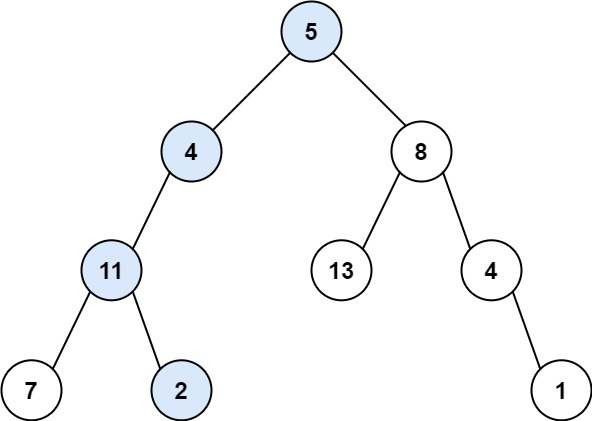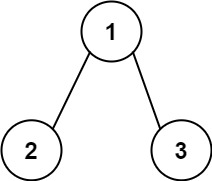## C# || How To Determine If Binary Tree Root To Leaf Path Sum Exists Using C#The following is a module with functions which demonstrates how to determine if a binary tree root to leaf path sum exists using C#.

1. Has Path Sum – Problem Statement

Given the root of a binary tree and an integer targetSum, return true if the tree has a root-to-leaf path such that adding up all the values along the path equals targetSum.

A leaf is a node with no children.

Example 1:``` Input: root = [5,4,8,11,null,13,4,7,2,null,null,null,1], targetSum = 22 Output: true ```

Example 2:``` Input: root = [1,2,3], targetSum = 5 Output: false ```

Example 3:

``` Input: root = [1,2], targetSum = 0 Output: false ```

2. Has Path Sum – Solution

The following is a solution which demonstrates how to determine if a binary tree root to leaf path sum exists.

``` 2. Has Path Sum - Solution C# // ============================================================================ // Author: Kenneth Perkins // Date: Oct 18, 2021 // Taken From: http://programmingnotes.org/ // File: Solution.cs // Description: Determines if a root to leaf path sum exists in a binary tree // ============================================================================ /** * Definition for a binary tree node. * public class TreeNode { * public int val; * public TreeNode left; * public TreeNode right; * public TreeNode(int val=0, TreeNode left=null, TreeNode right=null) { * this.val = val; * this.left = left; * this.right = right; * } * } */ public class Solution { public bool HasPathSum(TreeNode root, int targetSum) { return Search(root, targetSum); } public bool Search(TreeNode node, int targetSum) { if (node == null) { return false; } // Determine the current sum targetSum = targetSum - node.val; // Since this is a root-to-leaf check, evaluate for the // success condition when both left and right nodes are null if (node.left == null && node.right == null) { return targetSum == 0; } // Keep exploring along branches finding the target sum return Search(node.left, targetSum) || Search(node.right, targetSum); } }// http://programmingnotes.org/ 1234567891011121314151617181920212223242526272829303132333435363738394041424344 // ============================================================================//    Author: Kenneth Perkins//    Date:   Oct 18, 2021//    Taken From: http://programmingnotes.org///    File:  Solution.cs//    Description: Determines if a root to leaf path sum exists in a binary tree// ============================================================================/** * Definition for a binary tree node. * public class TreeNode { *     public int val; *     public TreeNode left; *     public TreeNode right; *     public TreeNode(int val=0, TreeNode left=null, TreeNode right=null) { *         this.val = val; *         this.left = left; *         this.right = right; *     } * } */public class Solution {    public bool HasPathSum(TreeNode root, int targetSum) {        return Search(root, targetSum);    }     public bool Search(TreeNode node, int targetSum) {        if (node == null) {            return false;        }         // Determine the current sum        targetSum = targetSum - node.val;         // Since this is a root-to-leaf check, evaluate for the        // success condition when both left and right nodes are null        if (node.left == null && node.right == null) {            return targetSum == 0;        }         // Keep exploring along branches finding the target sum        return Search(node.left, targetSum)            || Search(node.right, targetSum);    }}// http://programmingnotes.org/ ```

QUICK NOTES:
The highlighted lines are sections of interest to look out for.

The code is heavily commented, so no further insight is necessary. If you have any questions, feel free to leave a comment below.

Once compiled, you should get this as your output for the example cases:

``` true false false ```